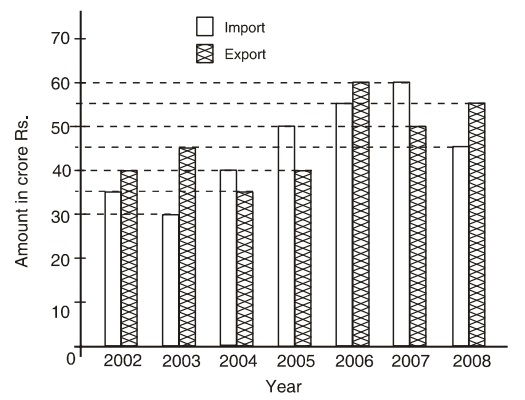Home » Data Interpretation » Bar graph » Question

#### Data Interpretation

Direction: Study the following graph carefully to answer the questions given below it.
Imports and Exports of spare parts by an automobile company over the given years.1. In which of the following pair of year and the percent increase in the export over the previous year is correctly matched?
1. 2002-03
2. 2004-5
3. 2005-06
4. 2007-08
##### Correct Option: D

In 2002-03, Total import = 35 + 30 = Rs. 65 crores
Total export = 40 + 55 = Rs. 95 crores
In 2004-05 . Total import = 40 + 50 = Rs. 90 crores
Total export = 35 + 40 = Rs. 75 crores
In 2005-06, Total import = 40 + 50 = Rs. 90 crores
Total export = 40 + 60 = Rs. 100 crores
In 2007-08, Total import = 60 + 45 = Rs. 105 crores
Total export = 50 + 55 = Rs. 105 crores
Hence, in 2007-08 pair of years the total import is equal to total export.